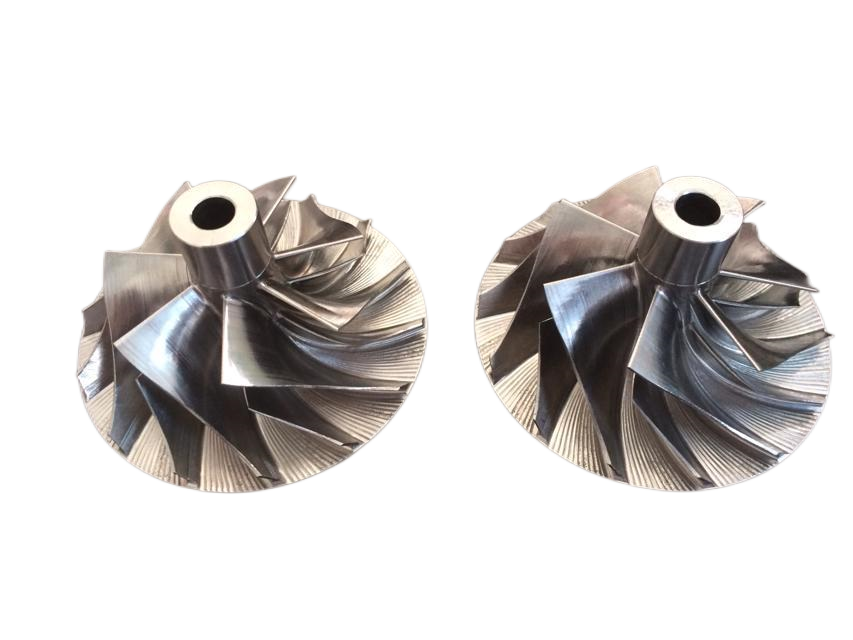# An error optimization method for CNC machining

## Wed Jul 13 16:16:38 CST 2022

1. A method for optimizing CNC machining errors, comprising the following steps: 1) acquiring real-time sampling data for each machine tool machining; 2) acquiring the part machining dimensional error data during each machine tool machining, and comparing it with the data obtained in step 1). The real-time sampling data of one machine tool processing is correspondingly stored; 3) the real-time sampling data of machine tool processing obtained in step 1) are processed respectively by using the time domain analysis method and the frequency domain analysis method to obtain the corresponding time domain characteristic parameters and frequency domain characteristic parameters, A feature parameter prediction model is established based on the mathematical fitting of the time-domain characteristic parameters and the frequency-domain characteristic parameters based on the least squares method, and the model is used to predict the time-domain characteristic parameters and the frequency-domain characteristic parameters, and the predicted values ​​of the time-domain characteristic parameters and the frequency domain characteristic parameters are obtained. The predicted value of the characteristic parameter in the domain; for the characteristic parameter in the time domain and the characteristic parameter in the frequency domain, a characteristic parameter prediction model is established based on the mathematical fitting of the least squares method. According to the processing sequence number 1, 2, 3, ..., i, the characteristic parameter a corresponding to each processing process is expressed as a1, a2, a3, ..., ai, for a1, a2, a3, . .., ai uses mathematical fitting based on the least squares method to obtain the "a-x" fitting curve, that is, the "a-x" fitting curve is the characteristic parameter prediction model, and the fitting work is completed by the relevant software; the above-mentioned relevant software uses, including but not limited to MATLAB completes the fitting work; 4) establishes a BP single hidden layer neural network model, using the time domain characteristic parameters and frequency domain characteristic parameters in step 3) and the part machining dimension error data obtained in step 2), for the BP single hidden layer The neural network model is trained, and the predicted value of the time domain feature parameter and the predicted value of the frequency domain feature parameter in step 3) are brought into the trained BP single hidden layer neural network model to obtain the predicted processing error; 5) According to step4 ), some programs are fine-tuned by the NC program optimization system to compensate for the machining error. 2. a kind of numerical control machining error optimization method according to claim 1, is characterized in that: described step 4) comprises the following steps: 41) establish BP single hidden layer neural network model; Wherein, BP single hidden layer neural network model Including input layer, hidden layer and output layer, its structure is N(s, p, q), where s is the number of neurons in the input layer, and the number of neurons in the input layer s and the number of selected feature parameters The same, p is the number of neurons in the hidden layer, and q is the number of neurons in the output layer; 42) Use the time-domain feature parameters and frequency-domain feature parameters in step 3) and the part machining dimension error data obtained in step 2) , train the BP single-hidden-layer neural network model; 43) bring the time-domain feature parameter prediction value and the frequency-domain feature parameter prediction value in step 3) into the trained BP single-hidden-layer neural network model to obtain the prediction processing error. 3. a kind of numerical control machining error optimization method according to claim 2 is characterized in that: in described step 42), it comprises the following steps: a) select time domain characteristic parameter and frequency domain characteristic parameter, and for selecting The eigenvalues ​​obtained by normalizing the determined feature parameters by the min-max method are used as the input layer input of the BP single hidden layer neural network model; b) The number p of neurons in the hidden layer is determined according to the empirical formula and Integer, where h is a constant, and 1≤h≤10; c) In the BP single-hidden layer neural network, the output neurons of the output layer use binary coding, and each group of binary codes corresponds to its corresponding error interval one-to-one; d) Each time the selected feature parameter input will recalculate the objective function value of the BP single hidden layer neural network model, obtain a new weight, and compare the objective function value with the expected target value: if the obtained objective function value is less than If the expected target value is determined, the weights are determined, and a BP single-hidden-layer neural network model that meets the requirements is obtained; if the obtained objective function value is not less than the expected target value, the gradient descent method is used to reverse the calculation, and the weights are adjusted layer by layer until it meets the requirements. Expected target value.